• 列出逻辑状态表分析图
千次阅读
2019-08-06 15:45:06

## 1.判定表驱动分析法

在之前介绍的因果图分析法也用到了判定表，但是没有详细介绍。判定表又称决策表，它是分析和表达多逻辑条件下执行不同操作的情况的工具。在黑盒测试中，基于判定表的测试是最具有逻辑性、最严格的测试。判定表可以将复杂的问题按照各种可能的情况列举出来，简单明了且避免疏漏。

测试思想：根据逻辑条件取值的组合所构成的多种情况，分别执行不同的操作，构造相应的测试用例。

决策表分类：

（1）有限项决策表：所有条件项的取值都是二值逻辑（True/False,，0/1...）

（2）扩展项决策表：所有条件项的取值有多个

决策表组成部分：

通常由以下4部分组成：

（1）条件桩—列出问题的所有条件

（2）条件项—针对条件桩给出的条件列出所有可能的取值

（3）动作桩—列出问题规定的可能采取的操作

（4）动作项—指出在条件项的各组取值情况下应采取的动作

具体案例分析见博客判定表驱动分析法设计测试用例

## 2.场景分析法

简单概念：

场景：用例场景用来描述流经用例的路径，从用例开始到结束遍历这条路径上的所有基本流和备选流。

基本流：经过用例最简单的路径

备选流：可能从基本流开始，在某个特定的条件下执行，再次加入到基本流里；或者源于一个备选流，从而终止用例而不再加入到某个流。

基本流和备选流的区分：

（1）基本流只有一个起点，一个终点；

（2）基本流是主流，备选流是支流；

（3）备选流可以始于基本流，也可以始于其它备选流

（4）备选流的终点，可以是一个流程的出口，也可以是回到基本流，还可以是汇入其它的备选流

（5）备选流汇合时，谁汇合到谁，取决于流量大小也即该流程出现的可能性大小，小的汇入大的

（6）如果在流程图中出现了两个不相上下的基本流，一般需要把它们分别当做一个业务看待。

详细案例可见博客场景分析法设计测试用例

### 3.流程分析法：

定义：流程分析法是把软件系统的某个流程看成路径，用路径分析的方法去设计测试用例。根据流程的顺序进行依次组合，使得流程的每个分支都可以走到。简单来讲，就是我们常见的流程图的方式来分析整个流程，再根据不同的路径执行不同的操作从而设计出测试用例。

详细可见博客流程分析法设计测试用例

### 4.状态迁移分析法：

步骤：

（1）根据需求明确状态节点

（2）绘制状态迁移图

（3）绘制状态迁移树

（4）抽取测试用例

详细的案例可见博客状态迁移分析法设计测试用例

### 5.错误推测法：

错误推测法是指测试人员依据自己的经验和直觉推测出程序可能出现问题的地方，从而有针对性的进行测试。它基于“某处发现了缺陷，则可能会隐藏更多的缺陷”这样的思想。在实际操作中，列出程序中所有可能的错误和容易发生的特殊情况，然后依据测试者经验作出选择。

错误推测法适用于我们进行经验测试，或者根据现有BUG进行拓展测试、用例补充。测试用例设计 判定表驱动分析 场景分析法 流程分析法
更多相关内容
• ## 组合逻辑电路的分析与设计

万次阅读 多人点赞 2020-01-17 21:36:47
1.组合逻辑电路的分析方法 首先给组合逻辑电路的一般分析方法，如1.1所示。 1.1 组合逻辑电路的一般分析方法 以下的组合逻辑电路为例。 ...

目录

1.组合逻辑电路的分析方法

2.公式化简和卡诺图化简

2.1公式化简的基本定律和规则

2.2逻辑函数的卡诺图化简法

2.3具有无关项的化简

3.竞争与冒险

3.1产生竞争-冒险的原因

3.2逻辑竞争与逻辑冒险

3.3逻辑冒险的识别与消除

3.3.1代数法

3.3.2卡诺图法

3.3.3逻辑冒险的消除

3.4功能竞争与功能冒险

3.5功能冒险的识别与消除

3.5.1功能冒险的识别

3.5.2功能冒险的消除

本文结合了原锆石科技文档资料(截取其中部分图表)，并参考了康华光《 电子技术基础 数字部分》（第六版）。

# 1.组合逻辑电路的分析方法

首先给出组合逻辑电路的一般分析方法，如图1.1所示。

图1.1 组合逻辑电路的一般分析方法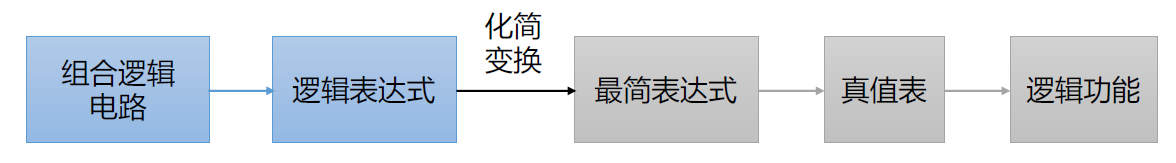以下图的组合逻辑电路为例。

图1.2 组合逻辑电路图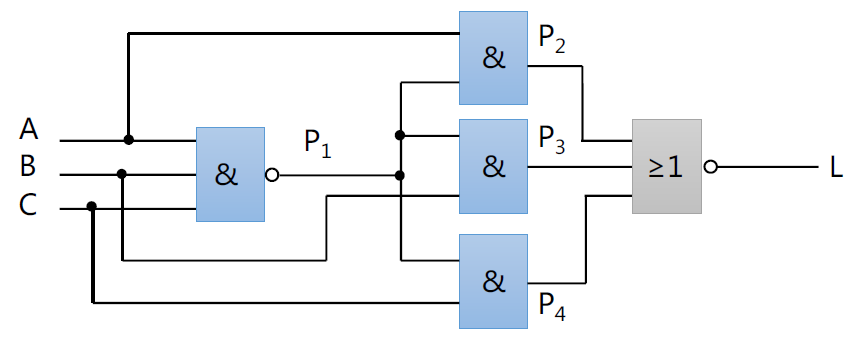（1）根据电路图写出逻辑表达式。由于现实中的电路图可能比较复杂，可以采取级联的分时分别写出各子模块的逻辑表达式。分别写出P1、P2、P3、P4的表达式。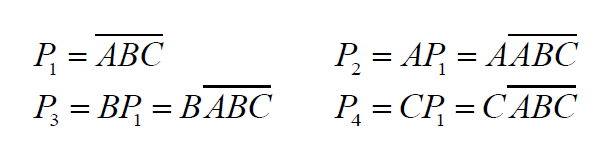得到最终的表达式：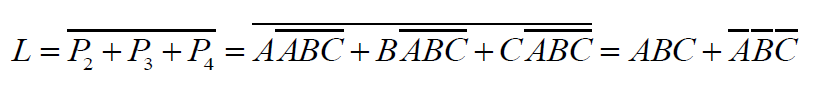（2）根据逻辑表达式写出真值表。

表1.1 真值表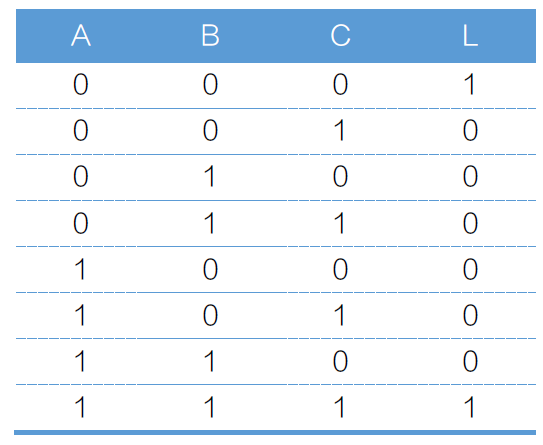（3）根据真值表分析电路的功能。如表1.1所示，当且仅当此电路当输入变量A、B、C全为0或全为1时，L输出为1；否则，L输出为0。

# 2.公式化简和卡诺图化简

## 2.1公式化简的基本定律和规则

表2.1.1是公式化简的九条基本定律。

表2.1.1 公式化简的九条基本定律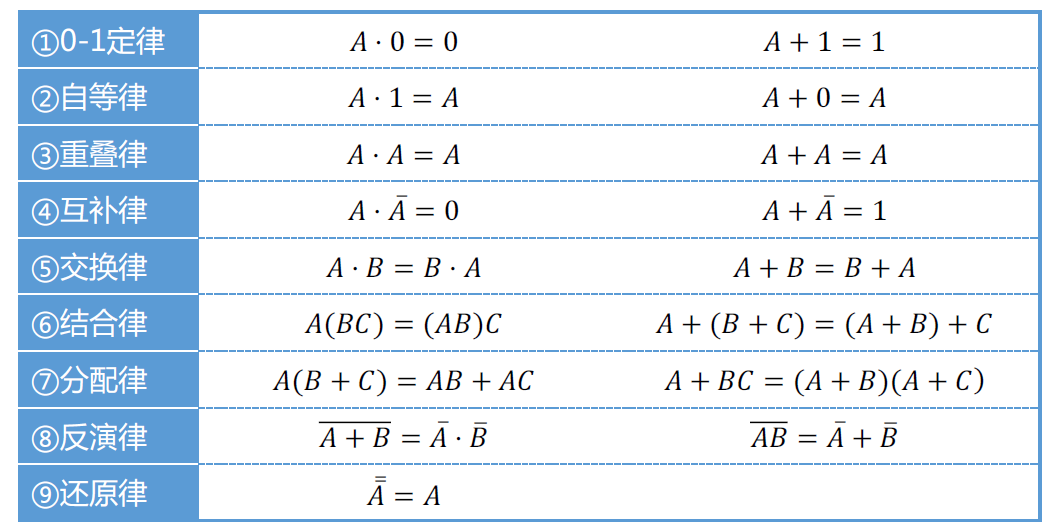逻辑代数除了上面的九条基本定律，还有三条重要的规则。分别是：代入规则、反演规则和对偶规则。

（1）所谓代入规则就是每个模块的输出都可以作其他模块的输入。已知等式，有函数，将代入前式中的，得到

（2）反演规则就是逻辑表达式中的运算符、常量和变量作如下变换：。例如，，则

（3）对偶规则。设是一个逻辑表达式，若把中的“与、或互换，0、1互换”，那么就得到一个新的逻辑表达式，这就是的对偶式，记作。例如，,则。变换时需注意保持原式中"先括号、然后与、然后或"的运算顺序。可以发现，公式化简的九条基本定律右边一列都可以由左边等式作对偶得到。将该规则概括为：当某个逻辑表达式相等，则它们的对偶式也相当

除了上述的九条基本定律和三条重要规则，还有一些公式化简的方法：

（1）并项法：利用公式，将两项合并成一项，并消去一个变量。例如，

（2）吸收法：利用公式，消去多余的项。根据代入规则，可以是任何一个复杂的逻辑式。例如，

（3）消去法：利用公式，消去多余的因子。例如，

（4）配项法：先利用增加必要的乘积项，再用并项或吸收的办法使项数减少。例如，

## 2.2逻辑函数的卡诺图化简法

关于卡诺图的引出以及卡诺图化简的依据这里不再赘述(感兴趣的可以参考电子技术书籍)，这里仅讨论卡诺图化简的一般步骤和实例。

在给出卡诺图化简得方法前，首先给出卡诺图的表示方法及其化简形式(以四变量为例)。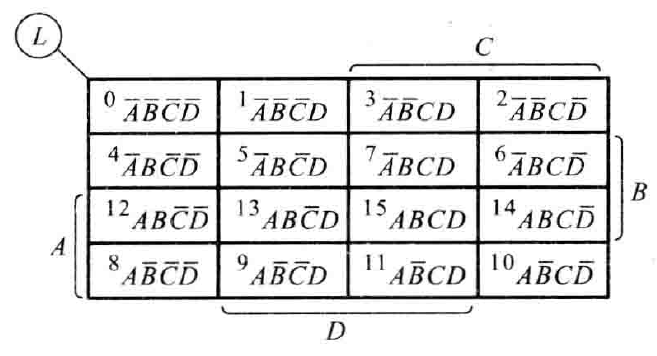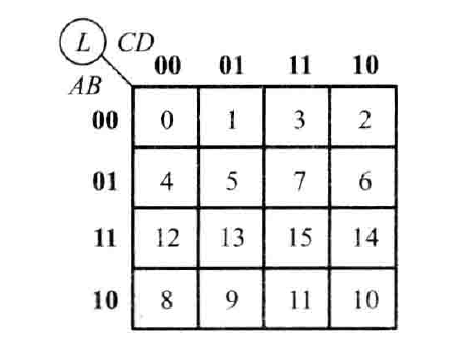将上述两幅卡诺图的表示方法对比可以发现，0000代表(表示次序依次为00，01，11，10，不要颠倒)​​​​​。

卡诺图化简的一般步骤如下：

（1）将逻辑函数写成最小项表达式；

（2）按最小项表达式填卡诺图(包含在式中的填1，其余填0)；

（3）圈出为1的相邻最小项，每个圈必须包含

（4）将所有包围圈对应的乘积项相加(每个包围圈的乘积项为变量的相同部分)。

注：在已知真值表的情况下，可将上述步骤的(1)(2)合并为一步

这里重点对画圈的原则进行说明：

（1）包围圈内的方格数必定是个；

（2）相邻方格包括上下底相邻，左右边相邻和四个角两两相邻

（3）同一个方格可以被不同的包围圈重复包围，但新增包围圈中一定要有新的方格(已有圈中的子集不可重新划定为圈，例如已圈定的4个方格大小的圈中的两个方格不可圈定为新圈)；

（4）包围圈内的方格要尽可能多(也就是说包围圈的数目要尽量少)，这决定了采用卡诺图化简后的表达式是否最简

例1 用卡诺图法化简下列逻辑函数，求的最简与-或表达式。

解：

（1）根据逻辑函数画出卡诺图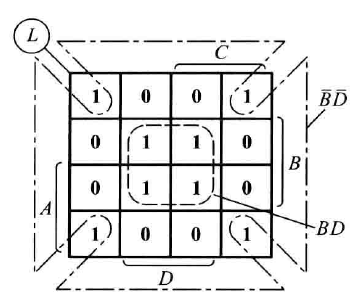（2）找出1的相邻最小项，圈出包围圈，合并最小项，得到最简与-或表达式

得到这两个乘积项的过程，这里再赘述一下(以中间的四格圈为例）。AB部分01和11中‘1’为共同元素，对应着B；CD部分01和11中‘1’为共同元素，对应着D，得到项。因此只需要先按行找他们的共同元素，再按列找共同元素

例2 化简下列逻辑函数

解：

（1）由画出卡诺图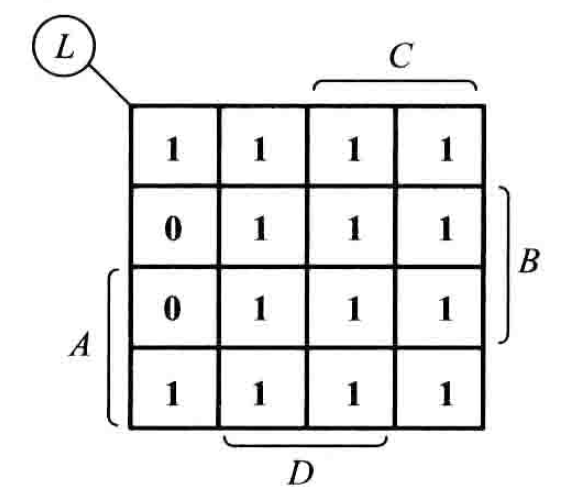（2） 卡诺图化简(这里由于1比较多，提供第二种思路)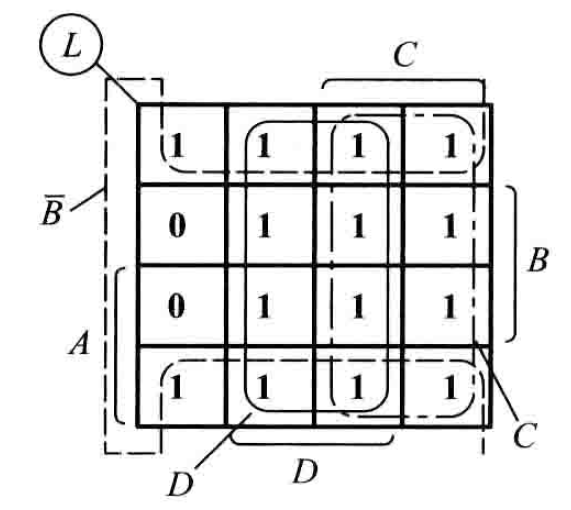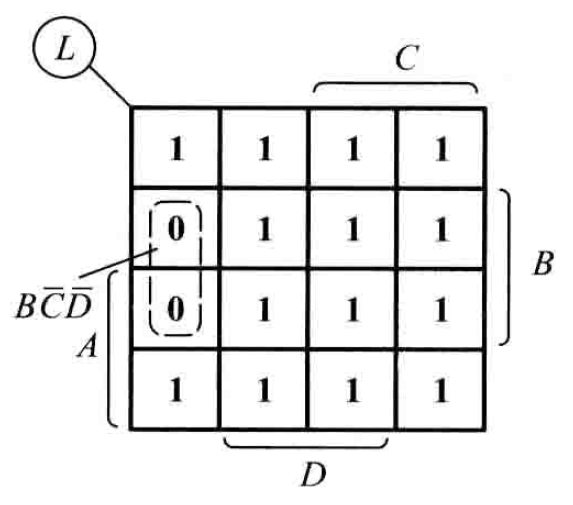法一（圈1） ：

法二（圈0）：，从而

## 2.3具有无关项的化简

除了上述这些具有确定逻辑表达式的卡诺图化简，在实际工作中，逻辑变量意味着特殊的现实意义，因此某些变量的取值组合并不会出现，也就是函数值可以是任意的（可以是0，也可以是1），变量取这些值所对应的最小项称为无关项任意项这点在由真值表进行卡诺图化简中体现得尤为明显

注：引入无关项的目的主要是为了化简原有的函数表达式，切勿画蛇添足，将额外的无关项圈出来（也就是说，例如把本来只可以圈画4个方格，引入无关项后可以圈画8个方格，但是不要单独将无关项直接圈画起来）

例：要求设计一个逻辑电路，能够判断1位十进制数是奇书还是偶数，当十进制为奇数时，电路输出为1；当十进制数为偶数时，电路输出为0。

解：

（1）写出真值表。用8421 BCD码表示十进制数，输入变量用A、B、C、D表示。1表示对应的十进制数为奇数，0表示对应的十进制数为0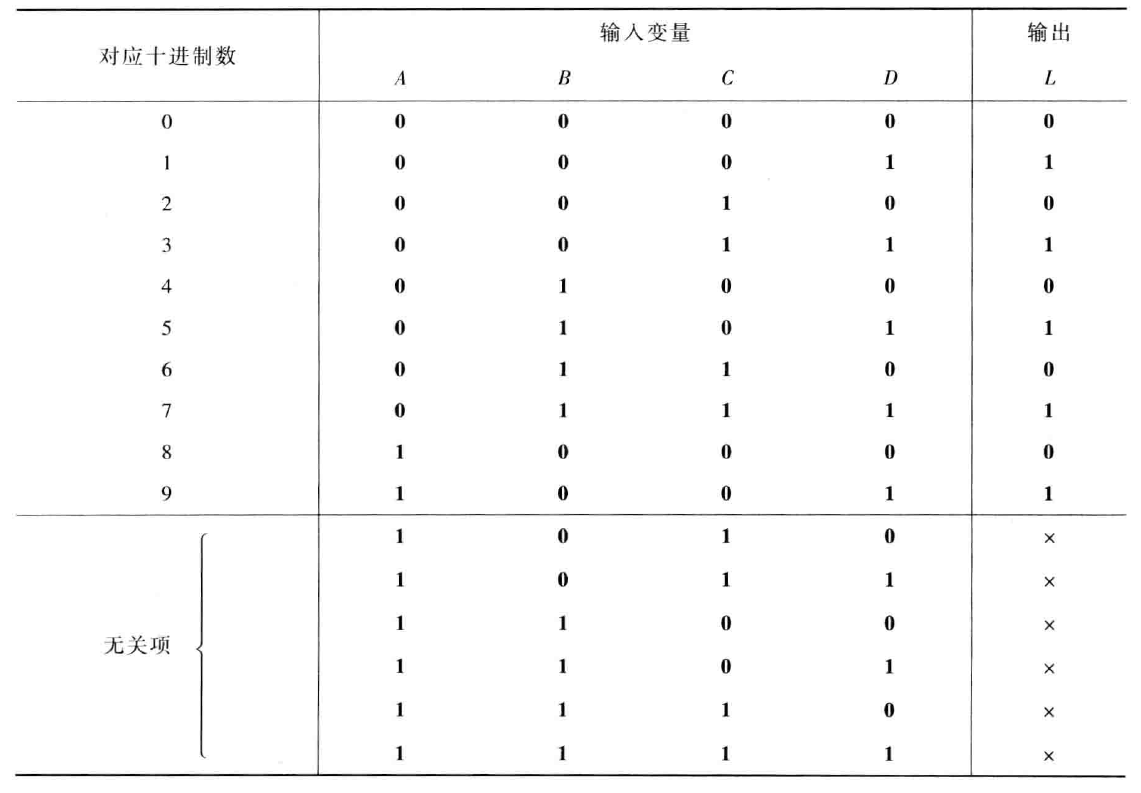注：下面出现的无关项是由于8421 BCD码只有十个数，表中的后六种组合式无关的。

（2）根据真值表，填写四变量卡诺图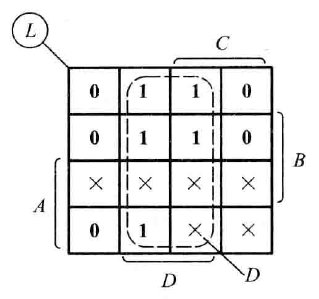（3）画包围圈，利用无关项，将最小项 对应的方格视为1，可以得到最大的包围圈，由此得到逻辑表达式

# 3.竞争与冒险

## 3.1产生竞争-冒险的原因

前面进行组合逻辑电路的分析与设计时，都没有考虑逻辑门的延迟时间对电路产生的影响(把门电路看成是理想门电路)，并且认为电路的输入和输出均处于稳定的逻辑电平。实际上，信号经过逻辑门电路都需要一定的时间。由于不同路径上门的级数不同，信号经过不同路径传输的时间不同。或者门的级数相同，而各个门延迟时间的差异，也会造成传输时间的不同。因此，电路在信号电平变化瞬间，可能与稳态下的逻辑功能不一致产生错误输出，这种现象就是电路中的竞争-冒险

根据其产生的原因不同，又可分为逻辑竞争、逻辑冒险和功能竞争、功能冒险。

## 3.2逻辑竞争与逻辑冒险

会导致"1型冒险"，会导致"0型冒险"

竞争就是在一个组合电路中，当某一个变量经过两个以上的路径到达输出端时，由于每条路径上的延迟时间不同，到达终点的时间有先后，这一现象被称为竞争，这里的竞争又可以称之为逻辑竞争。如下图所示：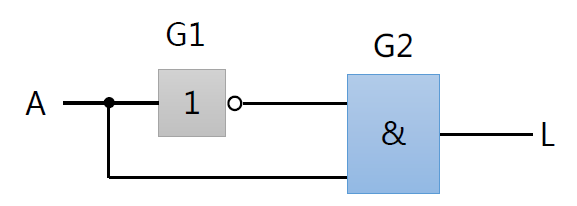输入端A的一端经过G1门，延迟了一个时间后到达G2门；而另一端直接连接在了G2门的输入端。因此，可以说同一个信号A，经过两条不同的路径分别到达G2门，并且由于它经过的两个路径的延迟时间不同，这两个信号到达输入端有先后关系，这样的现象称之为逻辑竞争。

冒险，就是在具有竞争现象的组合电路中，当某个变量发生变化时(0到1，1到0)，如果真指标所描述的逻辑关系或者功能受到短暂的破坏，在输出端出现一个本不该有的尖脉冲(毛刺)，这一现象称为逻辑冒险。逻辑冒险是一个错误的输出

由上图的电路图写出表达式，，然后再根据表达式写出真值表，如下图所示：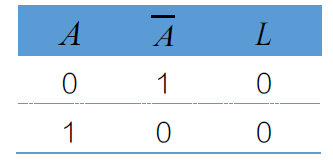从真值表可知，输出变量恒等于0，如下是其对应的理想波形图：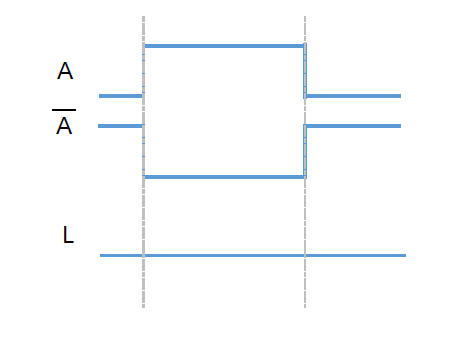然而，由于实际电路中门电路有延迟时间，将延迟时间记作，根据延迟时间画出实际状态的波形图，如下图所示：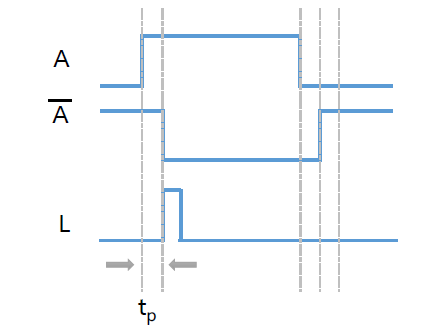从上图可以看出，信号经过一个G1非门，由于非门有一个延迟时间，所以不是另可变为0，而是经过一个延迟时间后才变为0。按照与逻辑功能，当=1，=0时，才等于1，但是不会直接立刻输出1，因为还要经过G2与门才会输出1。因此，这个电路的输出端也经过了一个延迟时间后才输出1(输出是由与经过非门得到的相与之后再经过一个非门的延迟时间后得到的，并且默认与门、非门延迟时间一致)。

在实际状态波形图中，出现这种短暂变为1的波形，相当于在电路中产生了一个尖脉冲，是不符合逻辑表达式要求的，是一个错误的波形。因此，将这种出现错误波形的现象称为逻辑冒险（逻辑冒险是由逻辑竞争导致的。又因为产生的是一个正的尖脉冲，所以通常称这种逻辑冒险为"1型冒险"。在波形的后部分，当变为0以后，不是立刻变为1，它要经过一个延迟时间后才会变成1，尽管在后面三条虚线内也出现了逻辑竞争，但是并没有出现逻辑冒险。由此说明，逻辑竞争并一定会导致逻辑冒险

"0型冒险"仅依次给出电路图、真值表和波形图，不再详细分析，如下图所示：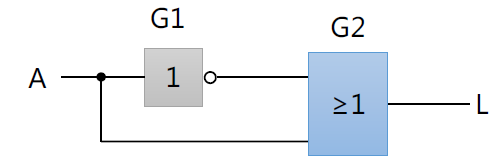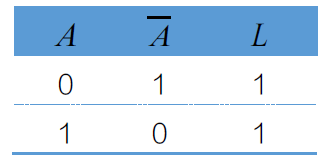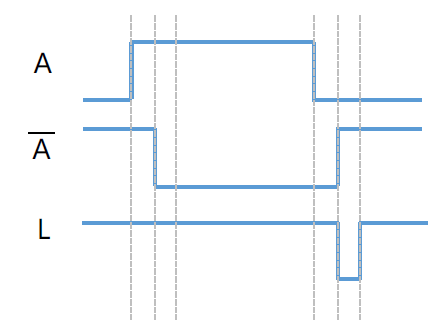## 3.3逻辑冒险的识别与消除

想要识别一个电路中是否存在逻辑冒险，通过有两种方法判别，一种是代数法，另一种是卡诺图法。

### 3.3.1代数法

代数法是根据电路图写出其逻辑表达式，在该逻辑表达式中某些逻辑变量取特定值0或1时，如果逻辑表达式能转换成，则该电路存在"1型冒险"；如果逻辑表达式能转换成，则该电路存在"0型冒险"。

以下图中的"0型冒险"为例：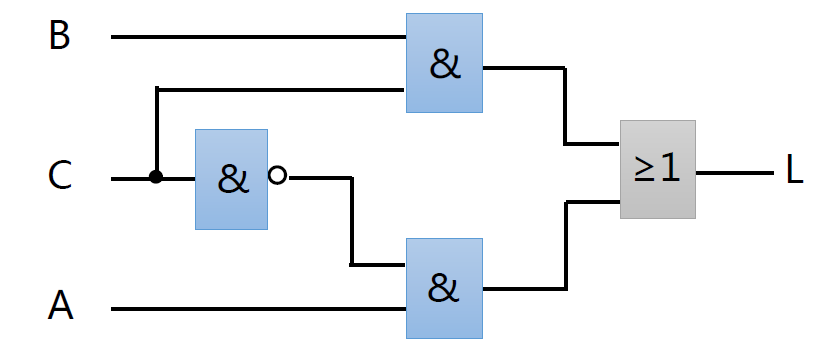由电路图可知，该电路的逻辑表达式为。在该逻辑表达式中，若输入变量，则有，因此可以断定该电路中存为"0型冒险"（识别"0型冒险"就是看能不能找到一个变量与其反变量进行"或操作"，"1型冒险"就是看能不能找到一个变量与其反变量进行"与操作"）。

### 3.3.2卡诺图法

卡诺图法是根据逻辑表达式画出卡诺图，在该卡诺图中，输入变量在卡诺图圈内改变时，输出不会有冒险现象；若输入变量是在相邻卡诺圈内的相邻处发生变化，并且是从一个卡诺图进入另一个卡诺圈，则可能产生逻辑冒险现象

为例进行说明，直接给出如下的卡诺图：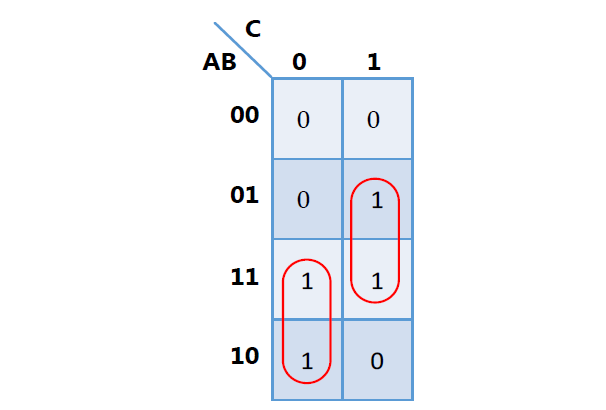从卡诺图可以看出，当由0→1时，此时的变化是在卡诺圈内变化，所以不会发生冒险。同理，当时，也是在卡诺圈内变化，因此不会出现冒险。但是，当由0→1时，相当于输出变量从110变化到111，此时的变化是从一个卡诺圈进入另一个卡诺圈，所以该电路存在冒险现象。

### 3.3.3逻辑冒险的消除

逻辑冒险可以通过电路的重新设计来消除，若在函数表达式中增加冗余项(相当于在卡诺图中增加多余的卡诺圈)，既不改变函数的逻辑功能，又可消除逻辑冒险，缺点是电路复杂度提高。以上述出现逻辑冒险的函数表达式为例，前面已经分析过，当时，出现逻辑冒险，因此只需要在原表达式中增加一个乘积项，表达式变为将不再产生冒险。由于在添加乘积项就，表达式不再是最简式，因此增加的乘积项也叫冗余项。通过卡诺图消除逻辑冒险的方法如下(增加卡诺圈)：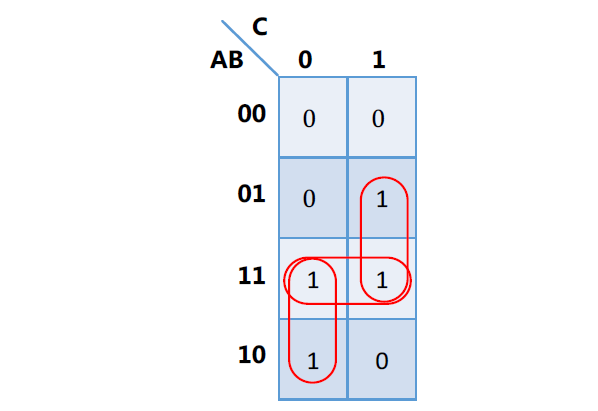## 3.4功能竞争与功能冒险

前面描述的逻辑竞争中输入变量只有1个，当多个变量经过两个以上的路径到达输出端时，同样会产生竞争，将这种竞争称为功能竞争，在具有功能竞争的组合电路中，也同样会产生冒险，将这种冒险称为功能冒险。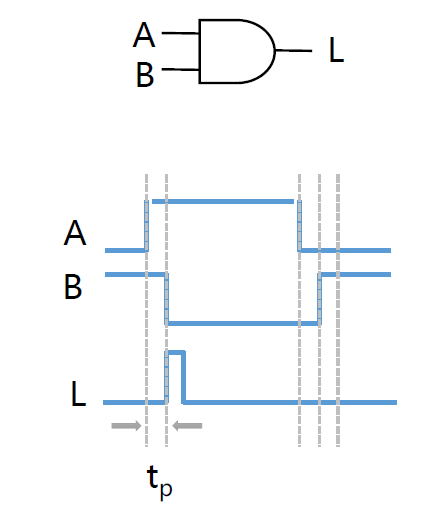对于与门，若稳态=1,=0或=0,=时1，输出 恒为0。但是在信号的传输过程中，由于传输时间的不同，造成在时间内，出现了一个错误的波形，将这种错误波形的现象称为功能冒险。又因为产生的是一个正的尖脉冲，所以称这种功能冒险为"1型冒险"。"0型冒险"类似，如下图：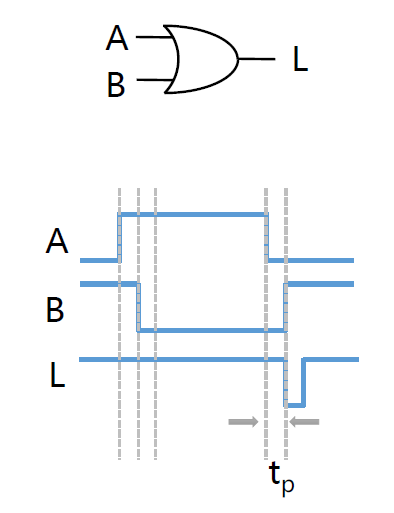注：逻辑竞争和功能竞争更多只是概念上的差别，逻辑冒险是由于同一个信号经过不同路径造成的，功能冒险是由于多个信号经过两个路径并最终形成的，本质上是一样的。

## 3.5功能冒险的识别与消除

### 3.5.1功能冒险的识别

功能冒险的识别通常采用卡诺图来进行判别，以下图为例: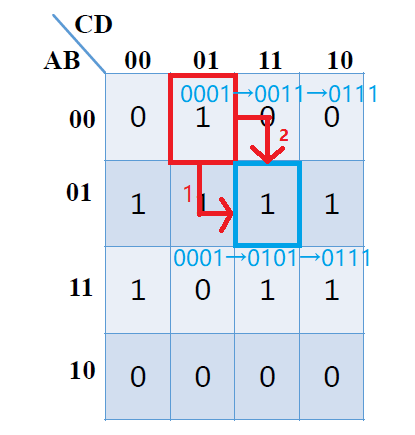根据卡诺图，可以知道时，；若，即(在这个过程中未发生变化)，则变化后函数的为稳定值也为1，由于输入变量实际上不可能同时变化，他们的变化总是有先有后的，若先变，则变化途径为，相应的输出变化为(图中红色箭头1变化过程)，此时没有发生冒险现象；但当先变时，的变化途径为，相应的输出变化为(图中红色箭头2变化过程)，因此出现了"0型冒险"(功能冒险产生于多变量发生变化，也就是它们不是左右、上下相邻关系)。

电路具有下列三个特点，则其输出可能产生功能冒险：

（1）有两个以上变量同时发生变化(如果仅有一个输出变量发生变化，则无功能冒险);

（2）变化前后，输出稳态值不变；

（3）在由n(n≥2)个变量发生变化的个方格中，既要有0，又要有1(如果对应个变量取值组合的输出值全为1或全为0，电路时不会产生功能冒险的)。

### 3.5.2功能冒险的消除

功能冒险时由于在输入n个变量同时变化时，它们的实际变化在时间上有先有后引起的，因此改变电路的逻辑设计并不能消除功能冒险。对待功能冒险常用的方法是增加选通信号或者在输出端并联放置滤波电容(电容能对窄脉冲起到平波的作用，消除输出端出现的逻辑错误，但同时也使输出波形上升或下降沿变得缓慢)

展开全文组合逻辑电路 卡诺图化简
• 题: 分析该时序逻辑电路的逻辑功能, 写电路的驱动方程、状态方程和输出方程, 画电路的状态转换, 并说明该电路是否可以自启动. 分析: 第一步, 根据电路逻辑图驱动方程和输出方程. 第二步, 根据驱动...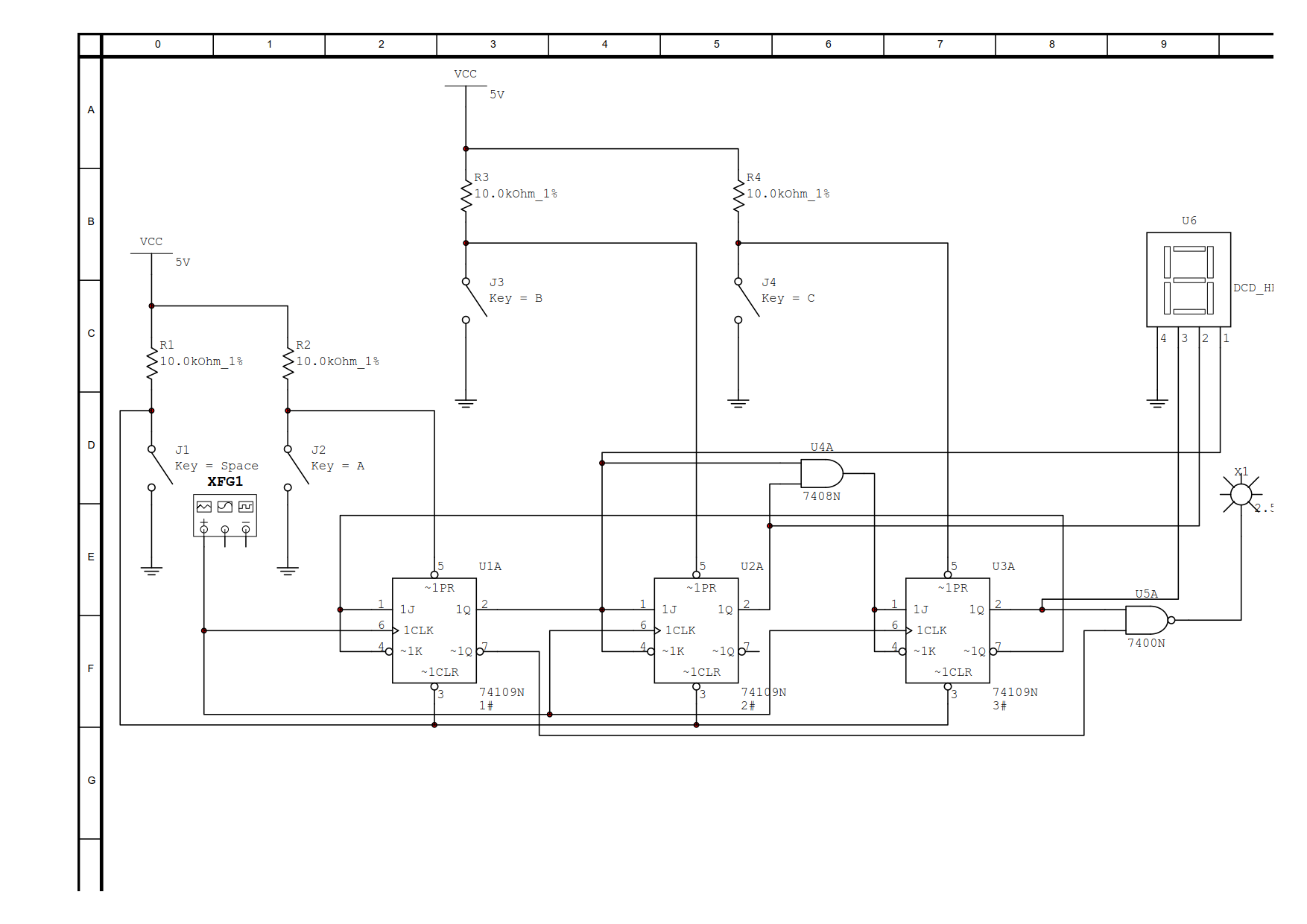题: 分析该时序逻辑电路的逻辑功能, 写出电路的驱动方程、状态方程和输出方程, 画出电路的状态转换图, 并说明该电路是否可以自启动.

分析:

第一步, 根据电路逻辑图写出驱动方程和输出方程.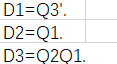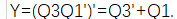第二步, 根据驱动方程写出状态方程.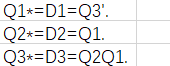第三步, 根据状态方程绘制状态表.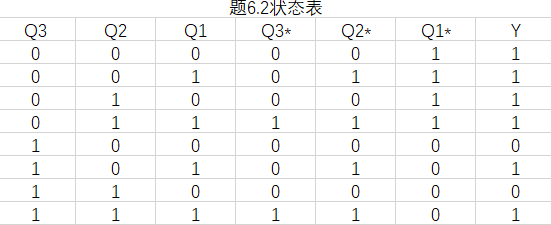第四步, 根据状态表绘制状态图.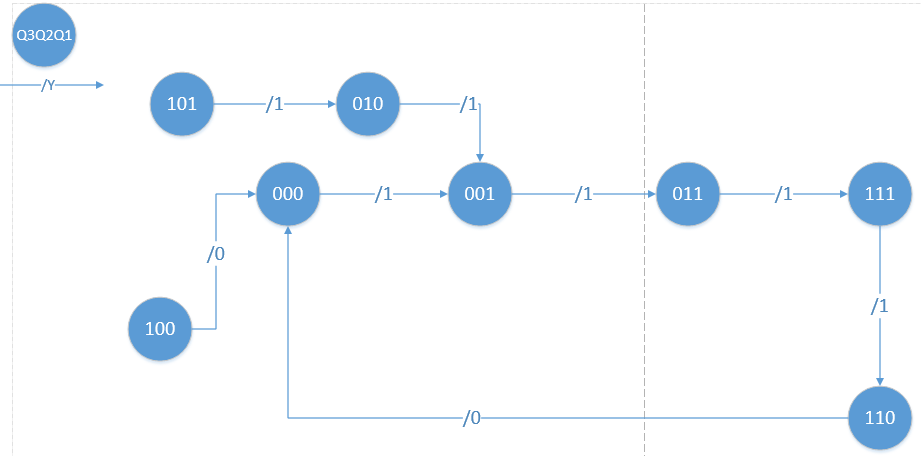第五步, 根据状态表分析电路的逻辑功能.

由绘制的状态表, 电路共有5个有效状态000、001、011、111、110; 当电路状态状态为110时, 输出Y=0, 其它状态的输出Y=1(提供下降沿脉冲进位). 所以, 这是一个同步五进制计数器.

电路的无效状态101、010、100都能进入有效循环中, 所以电路可自启动.

展开全文• 例题A(1) 写方程组(2) 根据方程组列出状态转移真值(3) 根据状态转移真值状态转移(4) 根据状态转移图画时序(5) 检查电路能否重启动(6) 画出完整的状态图(7) 判断电路功能3. 例题B(1) 写方程组(2) 列状态...

# 一、时序逻辑电路分析

## 1. 同步时序逻辑电路分析

### (1) 分析目的

分析时序逻辑电路在输入信号的作用下，其状态和输出信号变化的规律，进而确定电路的逻辑功能

### (2) 分析方法

利用驱动方程次态方程输出方程 ， 求出在任何给定的输入变量状态和存储电路状态下时序电路的输出和次态

• 驱动方程：触发器输入端的逻辑表达式
• 次态方程：将驱动方程代入对应触发器特征方程得到的逻辑表达式
• 输出方程 ：时序电路输出信号的逻辑表达式

### (3) 分析步骤

1.了解电路的组成：电路的输入、输出信号、触发器的类型等

2.根据给定的时序电路图，写出下列各逻辑方程式：

• 各触发器的驱动方程
• 各触发器的次态方程
• 时序电路的输出方程

3.列出状态转换表或画出状态图和波形图

4.确定电路的逻辑功能

## 2. 例题A

例1：分析以下电路的逻辑功能：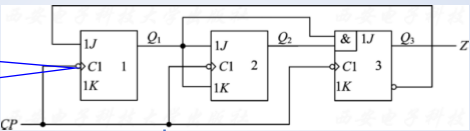### (1) 写方程组

• 输出方程： z = Q 3 n z = Q^n_3
• 驱动方程：
{ J 1 = Q 3 ‾ , K 1 = 1 J 2 = Q 1 n , K 2 = Q 1 J 3 = Q 1 n Q 2 n , K 3 = 1 \left \{ \begin{aligned} &J_1 = \overline {Q_3}, K_1= 1\\ &J_2 = Q^n_1, K_2 = Q_1\\ &J_3 = Q^n_1Q^n_2, K_3 = 1 \end{aligned} \right.
• 次态方程：这是下降沿触发的J-K触发器，因此 Q n + 1 = J Q n ‾ + k ‾ Q n Q^{n+1} = J \overline {Q^n} + \overline k Q^n

从而，可以得到以下的次态方程组：
{ Q 1 n + 1 = Q 3 n ‾   Q 1 n ‾ Q 2 n + 1 = Q 1 n Q 2 n ‾ + Q 1 n ‾ Q 2 n = Q 1 n ⊕ Q 2 n Q 3 n + 1 = Q 1 n Q 2 n Q 3 n ‾ \left \{ \begin{aligned} &Q^{n+1}_1 = \overline {Q^n_3}\ \overline {Q^n_1} \\ &Q^{n+1}_2 = Q^n_1\overline {Q^n_2} + \overline {Q^n_1}Q^n_2 = Q^n_1 \oplus Q^n_2\\ &Q^{n+1}_{3} = Q^n_1Q^n_2\overline {Q^n_3} \end{aligned} \right.

### (2) 根据方程组列出状态转移真值表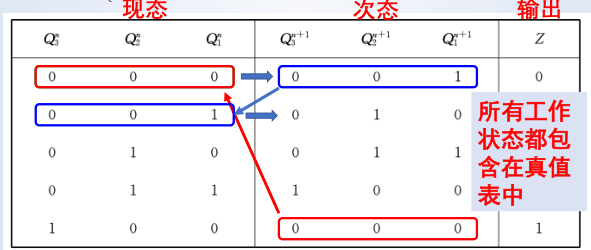### (3) 根据状态转移真值表画状态转移图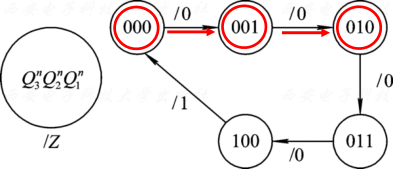### (4) 根据状态转移图画时序图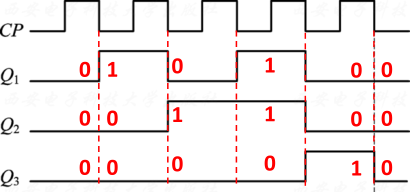### (5) 检查电路能否重启动

检查电路能否从 非工作状态 （未出现在状态转移真值表中的状态） 进入工作状态

非工作状态： 101 , 110 , 111 101,110,111
分别代入次态方程组和输出方程：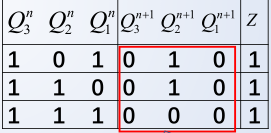电路可以由非工作状态进入工作状态，故可以自启！

### (6) 画出完整的状态图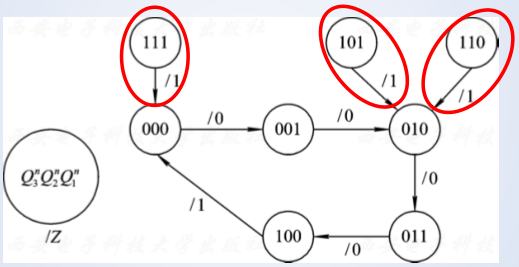### (7) 判断电路功能

由于时钟脉冲控制状态从 000 − 001 − 010 − 011 − 100 − 000 000-001-010-011-100-000 ，循环变换，逢五进一，可知，该电路是一个同步的五进制计数器

## 3. 例题B

分析以下电路的逻辑功能：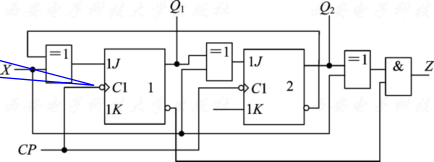### (1) 写方程组

这也是下降沿触发的J-K触发器。

• 输出方程： Z = ( X ⊕ Q 2 n ) ⋅ Q 1 n ‾ Z = (X \oplus Q^n_2) \cdot \overline {Q^n_1}
• 驱动方程：
{ J 1 = X ⊕ Q 2 n ‾ , K 1 = 1 J 2 = X ⊕ Q 1 n , K 2 = 1 \left \{ \begin{aligned} J_1 = X\oplus \overline {Q^n_2}, K_1 = 1\\ J_2 = X \oplus Q^n_1, K_2 = 1 \end{aligned} \right.
• 次态方程： Q n + 1 = J Q n ‾ + K ‾ Q n Q^{n+1} = J\overline {Q^n} + \overline K Q^n

可得次态方程组：
{ Q 1 n + 1 = ( X ⊕ Q 2 n ‾ ) Q 1 n ‾ Q 2 n + 1 = ( X ⊕ Q 1 n ) Q 2 n ‾ \left \{ \begin{aligned} Q^{n+1}_1 = (X \oplus \overline {Q^n_2}) \overline {Q^n_1} \\ Q^{n+1}_2 = (X \oplus Q^n_1)\overline {Q^n_2} \end{aligned} \right.

### (2) 列状态转移真值表

① 当 X = 0 X=0 时，化简方程：
{ Q 1 n + 1 = ( X ⊕ Q 2 n ‾ ) Q 1 n ‾ = Q 2 n ‾   Q 1 n ‾ Q 2 n + 1 = ( X ⊕ Q 1 n ) Q 2 n ‾ = Q 1 n Q 2 n ‾ Z = Q 2 n Q 1 n ‾ \left \{ \begin{aligned} &Q^{n+1}_1 = (X \oplus \overline {Q^n_2}) \overline {Q^n_1}=\overline {Q^n_2}\ \overline {Q^n_1} \\ &Q^{n+1}_2 = (X \oplus Q^n_1)\overline {Q^n_2} = Q^n_1 \overline {Q^n_2}\\ &Z = Q^n_2 \overline {Q^n_1} \end{aligned} \right.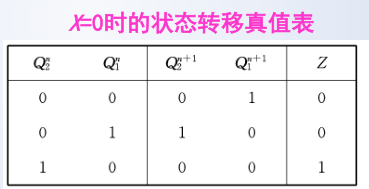② 当 X = 1 X=1 时，化简方程：
{ Q 1 n + 1 = ( X ⊕ Q 2 n ‾ ) Q 1 n ‾ = Q 2 n   Q 1 n ‾ Q 2 n + 1 = ( X ⊕ Q 1 n ) Q 2 n ‾ = Q 1 n ‾   Q 2 n ‾ Z = Q 2 n ‾   Q 1 n ‾ \left \{ \begin{aligned} &Q^{n+1}_1 = (X \oplus \overline {Q^n_2}) \overline {Q^n_1}= {Q^n_2}\ \overline {Q^n_1} \\ &Q^{n+1}_2 = (X \oplus Q^n_1)\overline {Q^n_2} = \overline{Q^n_1}\ \overline {Q^n_2}\\ &Z = \overline {Q^n_2}\ \overline {Q^n_1} \end{aligned} \right.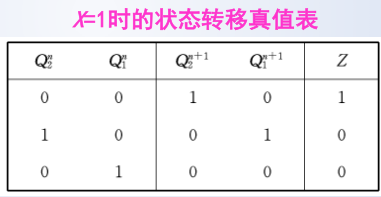### (3) 画状态转移图

X = 0 X =0 时的状态转移图：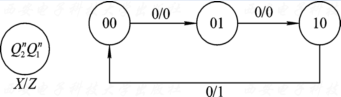X = 1 X =1 时的状态转移图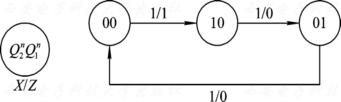### (4) 画时序图

Z = ( X ⊕ Q 2 n ) ⋅ Q 1 n ‾ Z = (X \oplus Q^n_2) \cdot \overline {Q^n_1}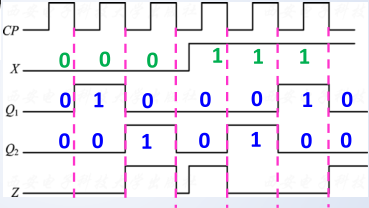### (5) 检查是否能自启

非工作状态： 11 11 ；分别代入次态方程组和输出方程，可得：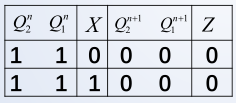该电路能够自启。

### (6) 画出完整的状态转换图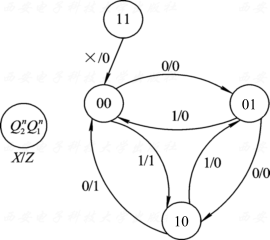### (7) 判断电路功能

该电路共有三个状态： 00 , 01 , 10 00,01,10
该电路是一个加减可控的三进制计数器

当时钟信号下降沿到来时：

• X = 0 X=0 ， 按照加 1 1 规律从 00 → 01 → 10 → 00 00→01→10→00 循环变化，并每当转换为 10 10 状态(最大数)时， 输出 Z = 1 Z=1
• X = 1 X=1 时， 按照减 1 1 规律从
10 → 01 → 00 → 10 10→01→00→10 循环变化，并每当转换为 00 00 状态(最小数)时， 输出 Z = 1 Z=1

## 4. 异步时序逻辑电路分析

### (1) 异步时序逻辑电路的特点

• 电路无统一的时钟信号；
• 除了时钟脉冲外，没有其它输入信号， 由时钟脉冲，直接引起电路状态的变化
• 由次态逻辑产生各触发器的驱动信号及时钟信号

### (2) 分析步骤

1.了解电路的组成：电路的输入、输出信号、触发器的类型等；

2.根据给定的时序电路图, 写出下列各逻辑方程式：

• 各触发器的时钟方程
• 各触发器的驱动方程
• 时序电路的输出方程
• 各触发器的次态方程

3.列出状态转换表、画出状态图和波形图
4.确定电路的逻辑功能

### (3) 注意

1.第一个触发器：每一次状态转换必须从输入信号所能触发的第一个触发器开始逐级确定
2.是否出现触发沿。逐个分析各触发器的时钟信号是否出现触发沿：

• 出现触发沿，求出该触发器的次态
• 未出现触发沿，记为 CP_n =0 ，该触发器保持原有状态

3.时间延迟
各个触发器的状态转换存在一定的延迟。只有当全部触发器状态转换完毕，电路才进入新的“稳定”状态，即次态

## 5. 例题C

分析以下电路的逻辑功能：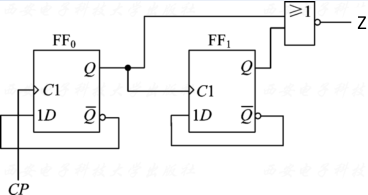### (1) 写方程组

电路基本情况：
• 采用两个上升沿触发 D D 触发器
时钟脉冲信号作为输入信号，不存在其他的输入信号
• 时钟 CP 的上升沿触发 F F 0 FF_0
Q 0 Q_0 0 → 1 0→1 时触发 F F 1 FF_1 ，要根据 Q 0 Q_0 的变化情况，确定是否更新 Q 1 Q_1 的次态

时钟方程：
{ C P 0 = C P ↑ C P 1 = Q 0 ↑ \left \{ \begin{aligned} &CP_0 = CP\uparrow \\ &CP_1 = Q_0 \uparrow \end{aligned} \right.

驱动方程组：
{ D 0 = Q 0 n ‾ D 1 = Q 1 n ‾ \left \{ \begin{aligned} &D_0 = \overline {Q_0^n} \\ &D_1 =\overline {Q_1^n} \end{aligned} \right.

输出方程： Z = Q 0 n + Q 1 n ‾ = Q 0 n ‾   Q 1 n ‾ Z = \overline {Q^n_0 + Q^n_1} = \overline {Q^n_0}\ \overline {Q^n_1}

由于 Q n + 1 = D Q^{n+1} = D ，次态方程组：
{ Q 0 n + 1 = D 0 = Q 0 n ‾ ⋅ C P 0 Q 1 n + 1 = D 1 = Q 1 n ‾ ⋅ C P 1 \left \{ \begin{aligned} &Q_0^{n+1} = D_0 = \overline {Q_0^n} \cdot CP_0 \\ &Q_1^{n+1} = D_1 = \overline {Q_1^n} \cdot CP_1 \end{aligned} \right.

计算过程：

• 将一组现态值带入次态方程组，计算出 Q 0 n + 1 Q_0^{n+1}
• 根据 Q 0 n → Q 0 n + 1 Q^n_0 \rightarrow Q_0^{n+1} 的变化情况，即时钟 C P 1 CP_1 的状态，确定是否计算更新 Q 1 n + 1 Q_1^{n+1} (时钟信号变化了)
• Q 0 n → Q 0 n + 1 : 0 → 1 Q^n_0 \rightarrow Q_0^{n+1} : 0 \rightarrow 1 ，则计算更新 Q 1 n + 1 Q_1^{n+1} ，否则 Q 1 n + 1 = Q 1 n Q_1^{n+1} = Q^n_1

### (2) 根据方程组列出状态转移真值表

只有在 Q 0 Q_0 处于上升沿时， Q 1 Q_1 才会变化：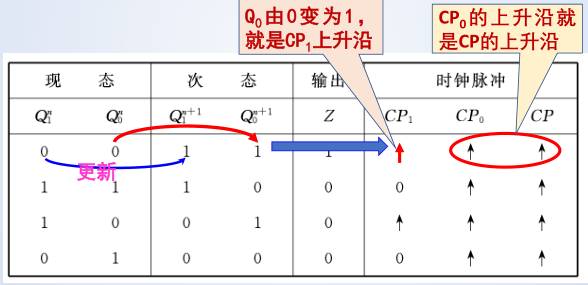### (3) 根据状态转移真值表画出状态转移图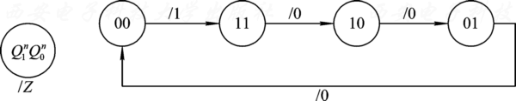### (4) 画出系统时序图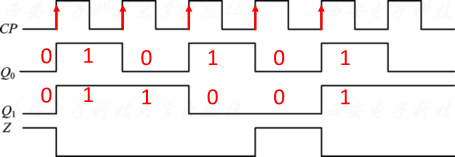### (5) 逻辑功能分析

该电路共有四个状态，在时钟脉冲作用下，按照减 1 1 规律循环变化： 00 → 11 → 10 → 01 → 00 00→ 11→ 10→ 01→00 ，是一个四进制减法计数器。其中， Z Z 借位信号

接下来就是时序逻辑电路的设计问题了。

# 二、时序逻辑电路的设计

时序逻辑电路的设计相对来说很有难度，这里只要求掌握同步时序电路的设计。

## 1. 同步时序逻辑电路的设计

同步时序逻辑电路的设计是分析的逆过程，其任务是根据实际逻辑问题的要求，设计出能实现给定逻辑功能的电路。

### (1) 同步时序逻辑电路设计的一般步骤

① 逻辑抽象：建立原始状态图和状态表；② 状态化简；③ 状态分配；
④ 选择触发器类型；⑤ 确定驱动方程组和输出方程；⑥ 检查自启动能力；⑦ 画出逻辑电路图

其中，状态化简和状态分配不要求掌握。

### (2) 逻辑抽象

• 确定输入、输出变量及电路的状态数
• 定义输入、输出逻辑状态和电路状态的含义
• 按题意建立原始状态转换图和状态转移真值表

#### a. 例1

设计一个五进制可逆计数器。当输入 x = 0 x=0 时，进行加 1 1 计数；当 x = 1 x=1 时，进行减 1 1 计数。

(1) 确定输入、输出变量及电路状态的数量

• 输入变量： 1 1
• 输出变量： 1 1
• 电路状态数量： 5 5

(2) 定义输入、输出变量及电路状态的含义

• 输入变量： x x —— 控制电路的功能选择
x = 0 x=0 ：加 1 1 计数
x = 1 x=1 ：减 1 1 计数
• 输出变量： y y —— 指示电路处于初始状态
y = 1 y=1 ：电路处于 S 0 S_0 状态
y = 0 y=0 ：电路处于其它状态
• 电路状态： S 0 − S 4 S_0-S_4 —— 五进制计数器的 5 5 种状态

(3) 列出状态转移真值表，画出状态转移图：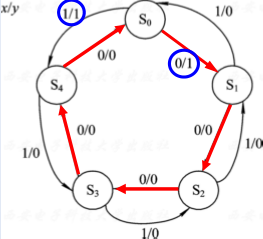红色箭头：加1计数状态转换顺序；黑色箭头：减1计数状态转换顺序.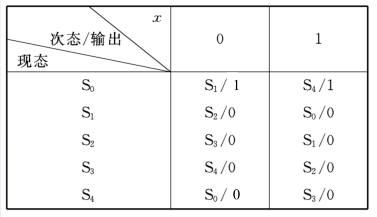#### b. 例2

设计一个串行数据检测器，在连续输入四个或四个以上的 1 1 时输出 1 1 ，否则输出 0 0

(1) 确定输入、输出变量及电路状态分析

• 输入变量： 1 1 个，表示输入数值串
• 输出变量： 1 1 个，表示检测结果
• 电路状态：
S 0 S_0 ：未输入 1 1
S 1 S_1 ：输入一个 1 1
S 2 S_2 ：连续输入两个 1 1
S 3 S_3 ：连续输入三个 1 1
S 4 S_4 ：连续输入四个或四个以上的 1 1

(2) 画出状态转移图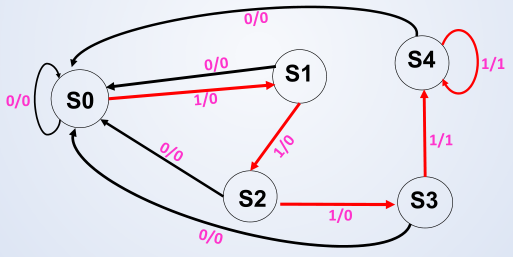(3) 列出状态转移表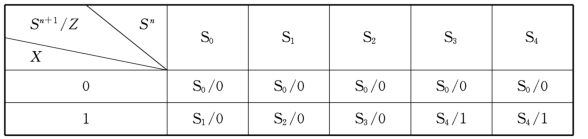### (3) 状态化简和分配

合并等效状态、 消去多余状态的过程，利用状态与状态间的等效关系来进行状态简化。

状态等效 ：
完全确定的状态表中，若对所有可能的输入序列 ，分别从某两个状态(假设为 S 1 S_1 S 2 S_2 )出发 ，所得到的输出序列都相同，且状态转移效果相同，则说这两个状态( S 1 S_1 S 2 S_2 )等效。记做：( S 1 S_1 S 2 S_2 )。此时 S 1 S_1 S 2 S_2 可以合并为一个状态。

等效的传递性：若有状态 S 1 S_1 S 2 S_2 等效，状态 S 2 S_2 S 3 S_3 等效，则状态 S 1 S_1 S 3 S_3 也等效，记为：( S 1 S_1 , S 2 S_2 )，( S 2 S_2 , S 3 S_3 ） → ( S 1 S_1 , S 3 S_3 )

等效类：所含状态都可以互相构成等效对的等效状态集合，即：
( S 1 , S 2 , S 3 ) (S_1,S_2 ,S_3) ( S 1 , S 2 ) (S_1, S_2) ( S 2 , S 3 ) (S_2, S_3) ( S 1 , S 3 ) (S_1, S_3)
( S 1 , S 2 ) (S_1, S_2) ( S 2 , S 3 ) (S_2, S_3) ( S 1 , S 3 ) (S_1, S_3) ( S 1 , S 2 , S 3 ) (S_1,S_2 ,S_3)

最大等效类：一个原始状态表中，不能被其它等效类所包含的等效类.

状态分配就是给最小化状态表中的每个状态分配一个二进制代码；
电路的状态是触发器状态的组合。如果电路有 N N 个状态， 2 n − 1 < N ≤ 2 n 2^{n-1} <N\le {2^n} ，则可用 n n 个触发器来实现.

### (4) 确定驱动方程组和输出方程

在确定了触发器类型的条件下，再确定驱动方程组。最常用的触发器是 D D 触发器，其次是 J − K J-K 触发器和 T T 触发器。

由状态转移表得到驱动方程组和输出方程的过程：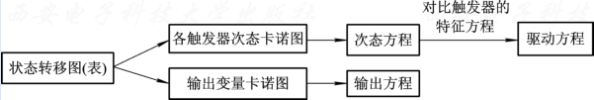#### c. 例3

分别用 D 触发器、J-K 触发器和 T 触发器实现下表所示的逻辑功能，试求对应的驱动方程和输出方程。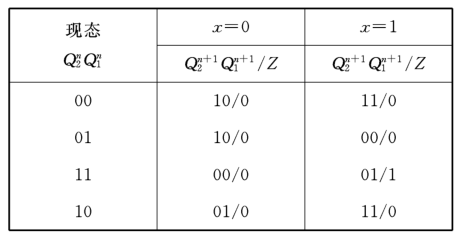(1) 绘制触发器次态卡诺图输出卡诺图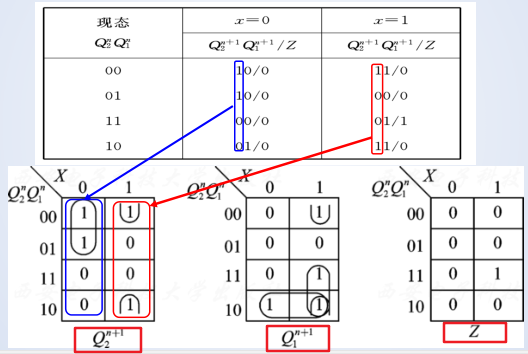(2) 求出次态方程和输出方程
{ Q 2 n + 1 = X ‾   Q 2 n ‾ + X Q 1 n ‾ Q 1 n + 1 = X Q 2 n + X Q 1 n ‾ + Q 2 n Q 1 n ‾ \left \{ \begin{aligned} &Q^{n+1}_{2} = \overline X\ \overline {Q^n_2} + X\overline {Q^n_1}\\ &Q^{n+1}_1 = XQ^n_2 + X\overline {Q_1^n} + Q^n_2\overline {Q^n_1} \end{aligned} \right.
输出方程： Z = X Q 2 n Q 1 n Z = XQ^n_2Q^n_1

(3) 确定驱动方程组和输出方程(将次态方程代入触发器的特征方程中)

• 若选用 D 触发器， Q n + 1 = D Q_{n+1} =D 。对比，得到
{ D 2 = X ‾   Q 2 n ‾ + X Q 1 n ‾ D 1 = X Q 2 n + X Q 1 n ‾ + Q 2 n Q 1 n ‾ \left \{ \begin{aligned} &D_{2} = \overline X\ \overline {Q^n_2} + X\overline {Q^n_1}\\ &D_1 = XQ^n_2 + X\overline {Q_1^n} + Q^n_2\overline {Q^n_1} \end{aligned} \right.

• 若选用 J-K 触发器， Q n + 1 = J Q n ‾ + K ‾ Q n Q^{n+1} = J\overline {Q^n} + \overline K Q^n ，可得：
Q 2 n + 1 = X ‾   Q 2 n ‾ + X Q 1 n ‾ = X ‾   Q 2 n ‾ + X Q 1 n ‾ ( Q 2 n ‾ + Q 2 n ) = ( X ‾ + X Q 1 n ‾ ) Q 2 n ‾ + X Q 1 n ‾ Q 2 n = ( X ‾ + Q 1 n ‾ ) Q 2 n ‾ + X Q 1 n ‾ Q 2 n Q 1 n + 1 = X Q 2 n + X Q 1 n ‾ + Q 2 n Q 1 n ‾ = X Q 2 n ( Q 1 n + Q 1 n ‾ ) + X Q 1 n ‾ + Q 2 n Q 1 n ‾ = ( X + Q 2 n + X Q 2 n ) Q 1 n ‾ + X Q 2 n Q 1 n = ( X + Q 2 n ) Q 1 n ‾ + X Q 1 n Q 2 n \begin{aligned} Q^{n+1}_{2} &= \overline X\ \overline {Q^n_2} + X\overline {Q^n_1}\\ &= \overline X\ \overline {Q^n_2} + X\overline {Q^n_1}(\overline {Q^n_2} + Q^n_2)\\ &= (\overline X + X \overline {Q^n_1}) \overline {Q^n_2} + X \overline {Q^n_1} Q^n_2 \\ &= (\overline X + \overline {Q^n_1}) \overline {Q^n_2} + X\overline {Q^n_1} Q^n_2\\ Q^{n+1}_{1} &= X {Q^n_2} + X\overline {Q^n_1} + {Q^n_2}\overline {Q^n_1}\\ &= X {Q^n_2} ( {Q^n_1} + \overline {Q^n_1})+ X\overline {Q^n_1} + {Q^n_2}\overline {Q^n_1}\\ &= (X +{Q^n_2} + X {Q^n_2}) \overline {Q^n_1} + X {Q^n_2} Q^n_1 \\ &= ( X + {Q^n_2}) \overline {Q^n_1} + X {Q^n_1} Q^n_2 \end{aligned}
从而：
{ J 2 = X ‾ + Q 1 n ‾ K 2 = X Q 1 n ‾ ‾ = X ‾ + Q 1 n { J 1 = X + Q 2 n K 1 = X Q 2 n ‾ = X ‾ + Q 2 n ‾ \left \{ \begin{aligned} J_2 &= \overline X + \overline {Q^n_1}\\ K_2 &= \overline {X \overline {Q^n_1}} = \overline X + {Q^n_1} \\ \end{aligned} \right.\\ \left \{ \begin{aligned} J_1 &=X + {Q^n_2}\\ K_1 &= \overline {X {Q^n_2}} = \overline X + \overline{Q^n_2} \\ \end{aligned} \right.

• 若选用 T 触发器， Q n + 1 = T Q n ‾ + T ‾ Q n Q^{n+1} = T\overline {Q^n} + \overline T{Q^n} ，这个就有一定难度了。为了求出驱动方程，我们分析如下：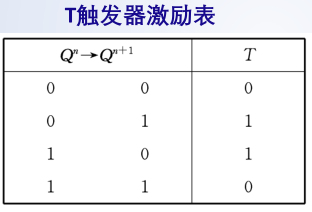T触发器要发生状态翻转，必须满足输入端信号 T = 1 T=1 ，若选用T触发器假设两个T触发器的输入端信号分别为T1和T2，由状态转移表画T1和T2的卡诺图：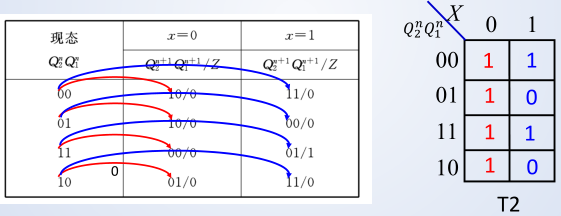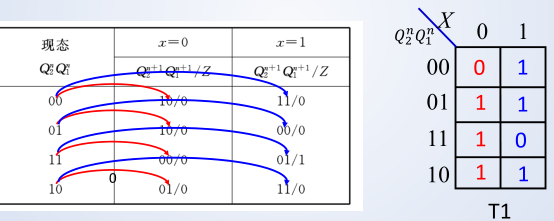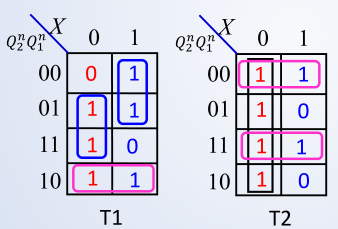对T1和T2的卡诺图进行化简，得到T1和T2的逻辑表达式：
{ T 2 = X ‾ + Q 1 n ‾   Q 2 n ‾ + Q 2 n Q 1 n T 1 = X ‾ Q 1 n + X Q 2 n ‾ + Q 2 n Q 1 n ‾ \left \{ \begin{aligned} T_2 &= \overline X + \overline {Q^n_1} \ \overline {Q^n_2} +Q^n_2Q^n_1\\ T_1 &= \overline {X} {Q^n_1} + X\overline{Q^n_2} + Q^n_2\overline {Q^n_1} \\ \end{aligned} \right.\\

### (5) 检查自启动功能

当所设计的电路中，触发器能表示的状态数大于有效状态数时，需要对所设计的电路进行实际工作状态讨论。

讨论两个问题 ：
(1) 电路万一进入无用状态，能否在时钟脉冲作用下进入有效状态，即是否具有自恢复功能和自启动功能
(2) 电路万一处在无用状态，是否会产生错误信号

一但出现以上两个问题都必须修改电路设计，否则将影响电路工作的可靠性和正确性。

#### d. 例4

J-K 触发器设计一个五进制同步计数器， 要求状态转移关系如右图所示。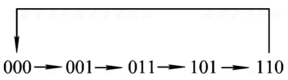解：

(1) 逻辑抽象，建立原始状态图和状态表
本例属于给定状态的时序电路设计问题，不存在逻辑抽象的问题，可以直接建立状态转移真值表。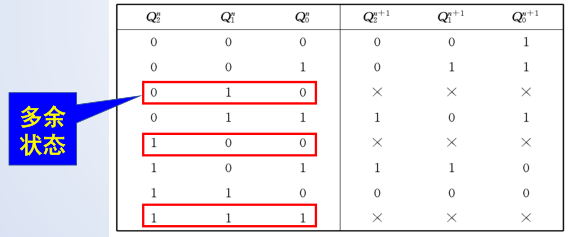(2) 选择触发器， 确定驱动方程和输出方程。根据状态转移真值表，画出各个次态的卡诺图。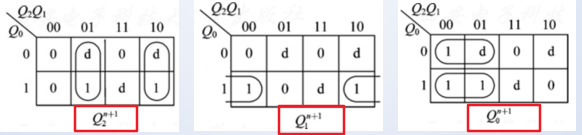若选用J-K触发器， 则由次态卡诺图求出其状态方程，再求出驱动方程
{ Q 2 n + 1 = Q 1 n Q 2 n ‾ + Q 1 n ‾ Q 2 n Q 1 n + 1 = Q 0 n Q 1 n ‾ Q 0 n + 1 = Q 2 n ‾ = Q 2 n ‾   Q 0 n ‾ + Q 2 n ‾ Q 0 n { J 2 = Q 1 n , K 2 = Q 1 n J 1 = Q 0 n , K 1 = 1 J 0 = Q 2 n ‾ , K 0 = Q 2 n \left \{ \begin{aligned} &Q^{n+1}_{2} =Q_1^n \overline {Q^n_2} + \overline {Q^n_1}Q^n_2\\ &Q^{n+1}_1 = Q^n_0 \overline {Q_1^n} \\ &Q^{n+1}_0 = \overline {Q^n_2} = \overline {Q^n_2}\ \overline {Q^n_0} + \overline {Q^n_2} Q^n_0 \end{aligned} \right. \left \{ \begin{aligned} &J_{2} =Q_1^n, K_2 = {Q^n_1}\\ &J_1 = Q^n_0, K_1 = 1\\ &J_0 = \overline {Q^n_2}, K_0 = {Q^n_2} \end{aligned} \right.

(3) 自启动检查：根据以上次态方程， 检查多余状态的转移情况。
状态转移表：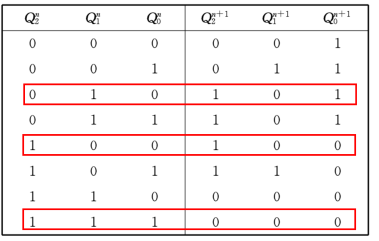状态转移图：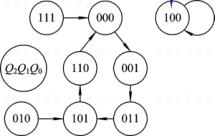存在孤立状态，因此不能自启。通过修改电路设计，可以避免不能自启动的问题。解决方法：改变卡诺图的圈法。如果希望能尽量使用任意项，那么只能对图( a )和图( c )的圈法作修改。 现选择对图( c )的圈法作修改，即仅改变 Q 0 Q_0 的转移，改进后的 Q 0 Q_0 卡诺图：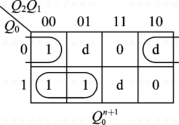{ Q 2 n + 1 = Q 1 n Q 2 n ‾ + Q 1 n ‾ Q 2 n Q 1 n + 1 = Q 0 n Q 1 n ‾ Q 0 n + 1 = Q 1 n ‾   Q 0 n ‾ + Q 2 n ‾ Q 0 n { J 2 = Q 1 n , K 2 = Q 1 n J 1 = Q 0 n , K 1 = 1 J 0 = Q 1 n ‾ , K 0 = Q 2 n \left \{ \begin{aligned} &Q^{n+1}_{2} =Q_1^n \overline {Q^n_2} + \overline {Q^n_1}Q^n_2\\ &Q^{n+1}_1 = Q^n_0 \overline {Q_1^n} \\ &Q^{n+1}_0 = \overline {Q^n_1}\ \overline {Q^n_0} + \overline {Q^n_2} Q^n_0 \end{aligned} \right. \left \{ \begin{aligned} &J_{2} =Q_1^n, K_2 = {Q^n_1}\\ &J_1 = Q^n_0, K_1 = 1\\ &J_0 = \overline {Q^n_1}, K_0 = {Q^n_2} \end{aligned} \right.
能够自启动的状态转移图：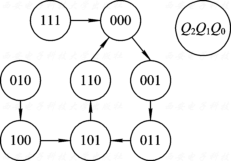(4) 画出电路图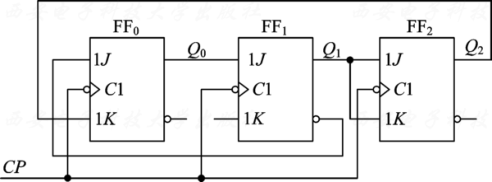# 三、练习题目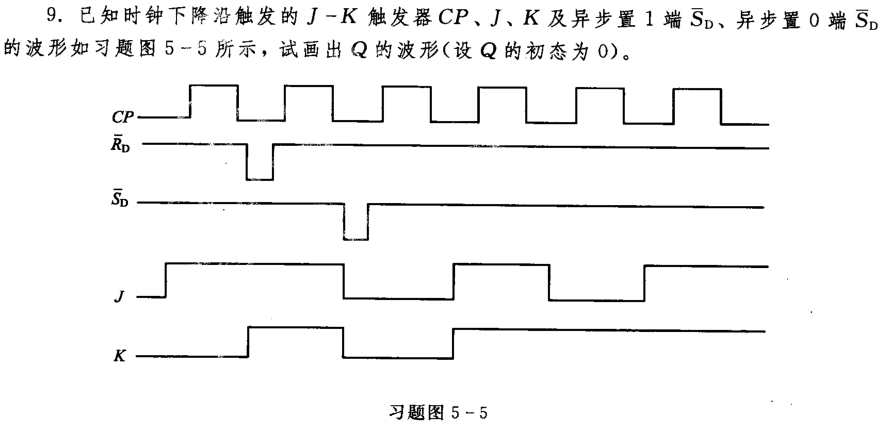这里题目出现了一定的错误，应该是异步置 0 0 R D ‾ \overline {R_D} ，异步置 1 1 S D ‾ \overline {S_D} 。当 S D ‾ = 1 , R D ‾ = 0 \overline {S_D} = 1, \overline {R_D} = 0 时， Q = 0 Q = 0 ；当 S D ‾ = 0 , R D ‾ = 1 \overline {S_D} = 0, \overline {R_D} = 1 时， Q = 1 Q = 1 。因此 Q Q 的波形图如下：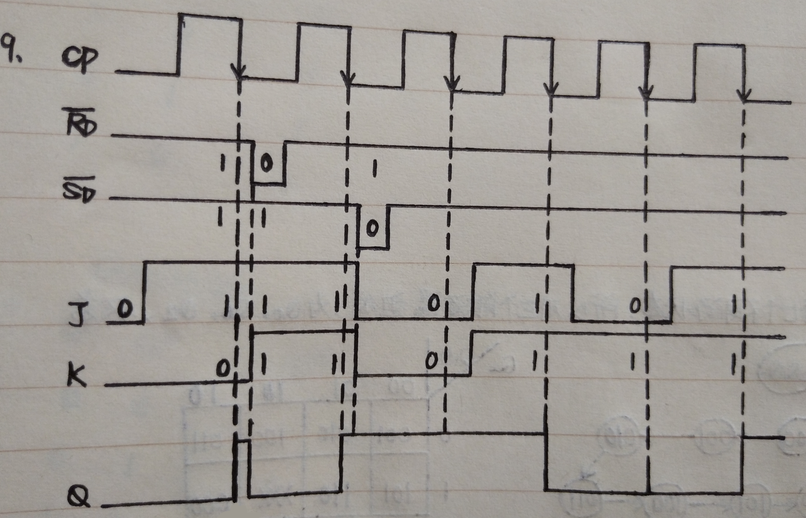看了答案，将答案更新如下。有的地方我没有写完整，有的地方我和答案不太一样，但是应该是对的。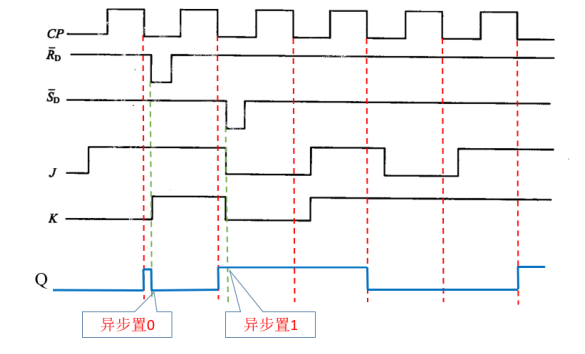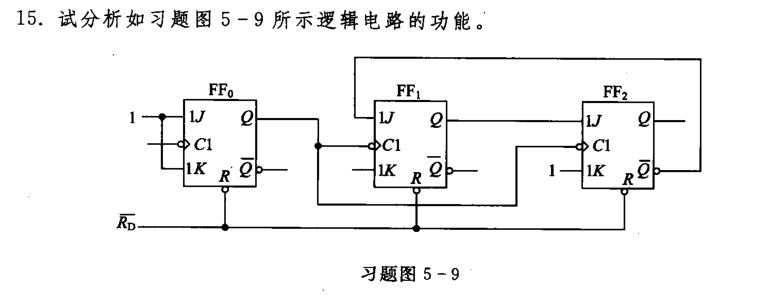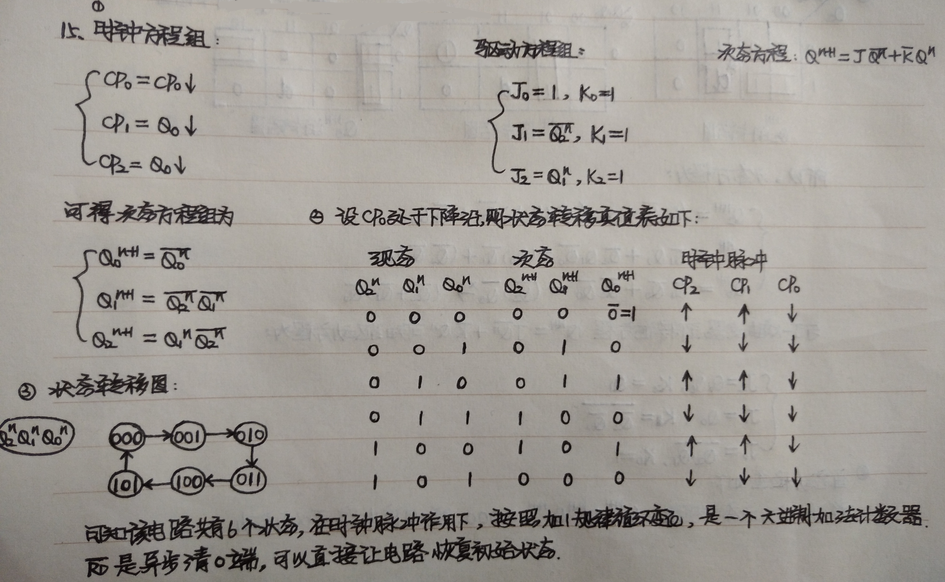上面没有检查自启动：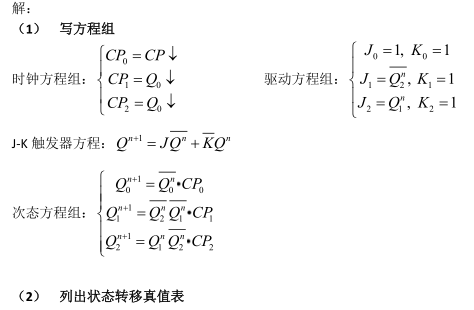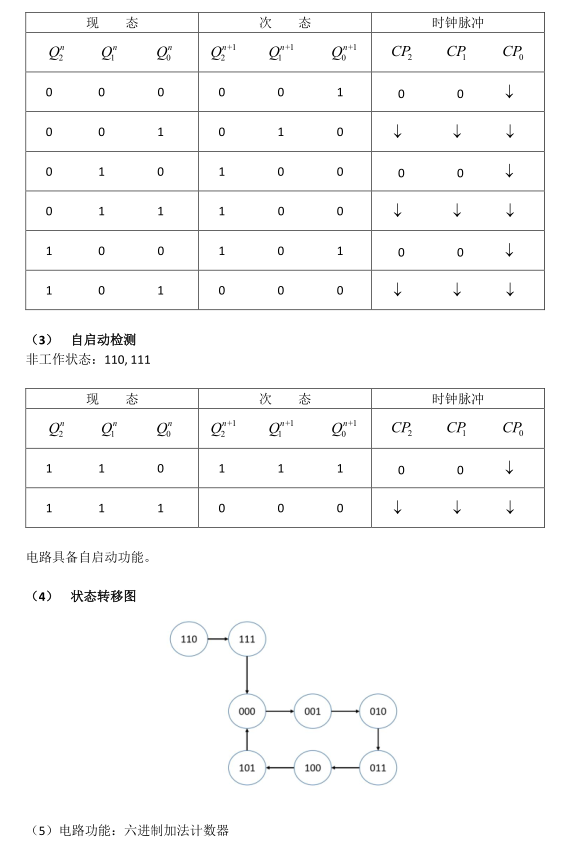17. 17. J − K J-K 触发器和门电路设计一个七进制加法计数器。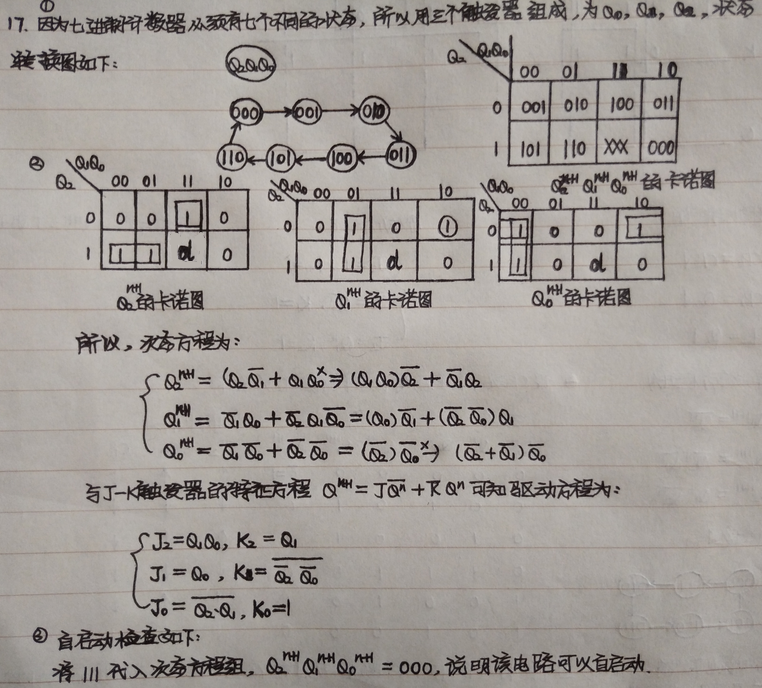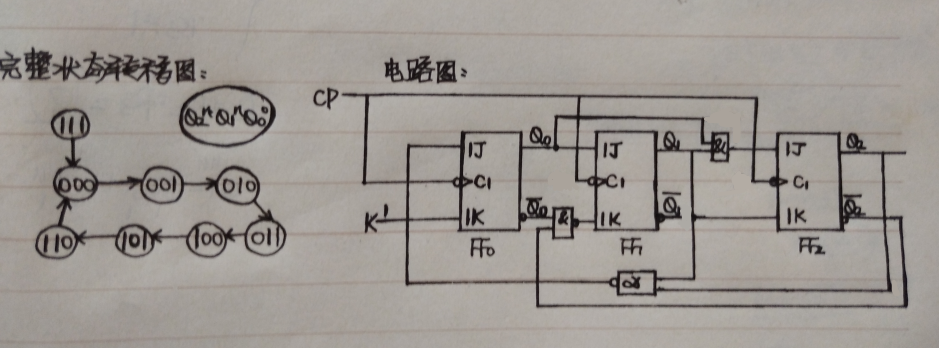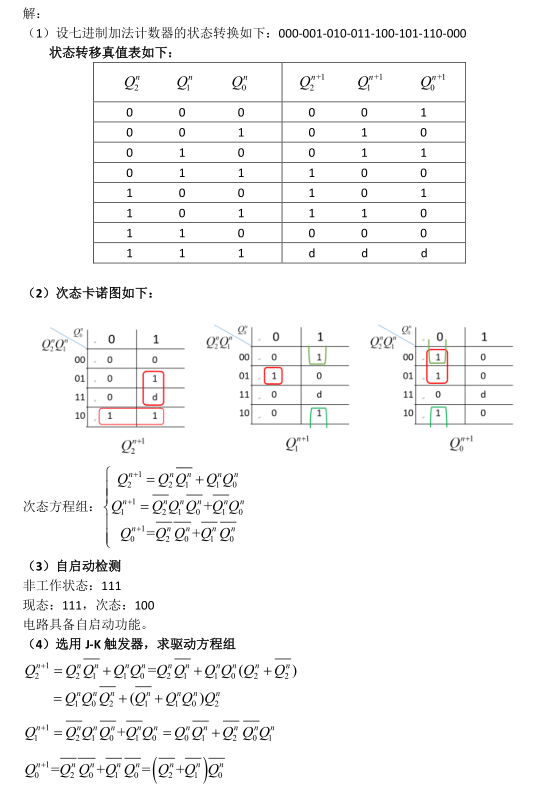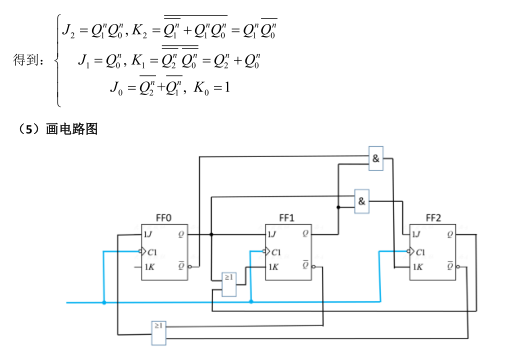展开全文• 数字电子技术基础：第六章 时序逻辑电路的分析与设计.ppt
• 同步时序电路分析的“核心”——借助触发器的新状态（次态）表达式列出时序电路的状态转换或画出状态转换。 同步时序逻辑电路分析的一般步骤 驱动方程代入JK触发器的特性方程。 不断循环。 电路没有输入。 ...
• 专题一：时序逻辑电路的分析和设计 说明：由于CSDN文本限制，反变量均写为X（注意X前面有）；内容、图片可能不完全显示，原版word文档请点击更多文章。 链接：https://pan.baidu.com/s/1jJhtwqE 密码：w208 ...计算机专业课 时序逻辑电路分析
• 组合逻辑电路在逻辑功能上的特点是任意时刻的输出仅仅取决于该时刻的输入，与电路原来的状态无关。而时序逻辑电路在逻辑功能上的特点是任意时刻的输出不仅取决于当时的输入信号，而且还取决于电路原来的状态，或者说...
• ## 时序逻辑电路的设计与分析

万次阅读 多人点赞 2020-03-26 16:58:57
1.时序逻辑电路的基本结构与分类 1.1时序电路的基本结构 时序电路的基本结构如下所示，它由
• 时序逻辑将会是本笔记的最后几章的主题。虽然数字电路课程还包括脉冲电路、模数转换、EDA等内容，但那些和本文的主线内容（不注重硬件搭建的电路设计）关系就不大了。一、时序功能我们从一个例子开始，说明时序逻辑...
• 试求下图示电路的驱动方程、状态方程, 并画出状态表. 再在本电路的基础上, 修改反馈逻辑, 将电路改造成同步八进制计数器. 根据上述电路, 可求得 D0=Q2Q1+Q1Q0'+Q2Q0', D1=Q1异或Q0, D2=Q2Q1'+Q2Q0'+Q2'Q1Q0. ...同步时序逻辑电路设计
• ## 数字逻辑笔记

千次阅读 2021-12-08 20:37:19
数字逻辑与数字系统设计 边写每章作业边记的解题方法……应付考试用
• ## 需求分析、ER图、状态转换图

千次阅读 多人点赞 2020-05-06 17:40:35
一、软件需求分析 基本任务： 系统必须做什么？ 确定系统必须完成哪些工作，也就是对目标系统尽可能提出完整、准确、清晰、具体的要求。 写软件需求规格说明书，以书面形式准确地描述软件需求。 为什么要进行需求...
• ## 因果图分析法

千次阅读 2021-08-24 16:14:12
目录 一、因果图法 1.理解 二、因果需要掌握的基本知识 ...因果是一种简化了的逻辑图，能直观的表明程序输入条件（原因）和输出动作（结果）之间的相互关系； 因果图法是借助图形来设计测试黑盒测试 测试类型 测试工程师
• T触发器的特性反相器 单稳态 图略 6.26分析图P6.26所示的电子门铃电路，当按下按钮S时可使门铃鸣响。 (1)说明门铃鸣响时555定时器的工作方式。 (2)改变电路中什么参数能改变铃响持续时间？ (3)改变电路中什么参数...
• 6.1 概述一、时序逻辑电路的特点 功能上：任一时刻的输出不仅取决于该时刻的输入，还与电路原来的状态有关。 例：串行加法器，两个多位数从低位到高位逐位相加 ...分析：找给定时序电路的逻辑功能
• 文章目录A 组合逻辑电路的分析和设计方法A.a 组合逻辑电路的特点及描述A.b 组合逻辑电路的分析A.c 组合逻辑电路的门级电路设计A.d 组合逻辑电路中的竞争-冒险现象 A 组合逻辑电路的分析和设计方法 A.a 组合逻辑电路...
• 什么逻辑库、物理库？ 逻辑库/逻辑文件：给用户看的（即Database和Table就是我们常说的逻辑库的范畴） 物理库/物理文件：存储在计算机中的（即机器和Port就是我们常说的物理库的范畴。） 一个服务器有多个实例(port...
• ## 软件工程 第六章：状态图

万次阅读 多人点赞 2019-01-19 21:08:43
状态图定义：状态图描述了一个对象或交互过程在它的生命周期中对**一系列外界激励的所呈现的不同状态以及它相应的响应和活动。**状态图描述了一个状态机。 状态机定义：根据当前事件,以及对以前事件的响应的结果...软件工程 状态图
• 第5章 时序逻辑电路习题 时序逻辑电路的分析就是在给定逻辑电路的基础上列出电路的输出方程驱动方程和状态方程得出状态转换或时序从而分析电路的逻辑功能 其步骤如下 1由所给的逻辑电路写出输出方程驱动方程 2...
• ## 时序逻辑3

千次阅读 2020-05-09 00:01:45
一、同步时序逻辑分析 1、概述 (1)、时序逻辑电路特点 电路任一时刻的输出状态不仅取决于当时的输入信号，而且还取决于电路原来的状态，即与以前的输入有关 (2)、时序逻辑电路结构 ①、米里型时序电路：输出与输入...时序逻辑电路分析 时序逻辑电路设计
• 组合逻辑电路一、组合逻辑电路的分析二、组合逻辑电路的设计设计组合逻辑电路需要注意的问题三、组合逻辑电路中的竞争冒险产生原因消除方法四、计算机体系结构涉及的组合逻辑电路编码器译码器/数据分配器数据选择器...
• 1 时序逻辑电路 1.1 介绍 输出往往反馈到输入端，与输入变量一起决定电路的输出状态。 1.2 特点 任意时刻输出不仅取决于该时刻输入变量的状态，而且还与原来的状态有关。 1.3 锁存器 1.3.1 特点 有两个互补的输出...
• 主要有组合逻辑电路分析题目（逻辑表达式、列出真值、写出逻辑功能）时序电路分析题（写出输出方程组、激励方程组和次态方程组表达式、列出状态图、确定逻辑功能）逻辑电doc文档
• 学会逻辑分析仪的使用方法。实验原理数字电路分为组合逻辑电路和时序逻辑电路两类。任意时刻电路的输出信号仅取决于该时刻的输入信号，而与信号输入前电路所处的状态无关，这种电路叫做组合逻辑电路...
• 生存分析定义 随访研究（follow-up study）是一种前瞻性研究（prospective study） 随访研究的研究设计可以是横断面研究（cross sectional study）、病例对照研究（case-control study），也可以是队列研究（cohort ...
• ## 电商订单逻辑图

万次阅读 多人点赞 2018-11-27 13:09:04
想要涉及好这一块内容，需要根据目前自己公司的业务情况，列出所支持的优惠类型，再枚举出各种组合下的优惠类型，才能保证流程的完整性。 时间信息 ：记录各个卡点下的时间，一是记录，二也是方便售后验证和客户...
• 1. 分析与设计同步时序逻辑电路的分析方法时序逻辑电路的分析，就是从给定的时序电路的逻辑电路出发，分析得 到其逻辑功能。具体讲，就是确定电路的输入和现态如何决定了电路的输出和次态，从而得到电路的状态迁移...
• 逻辑性 从事软件开发行业的同学们或多或少都具有相当不错的逻辑性，毕竟编程开发本身就是逻辑性较强的任务。但是大家是否考虑过这种逻辑性应该怎么应用到社交技巧上？下面就跟着笔者一起来详细分析下吧。 逻辑思维...逻辑思维 思考问题
• ## 触发器真值表

千次阅读 2020-12-19 04:19:38
任务1触发器电路一、实验目的1、掌握D触发器、JK触发器等基本...设计其他如RS触发器，并分析它们相互转化的方法3、通过模拟和仿真，分析和验证各种出发器的逻辑功能及其触发方式三、实验要求1、写D触发器、JK触......